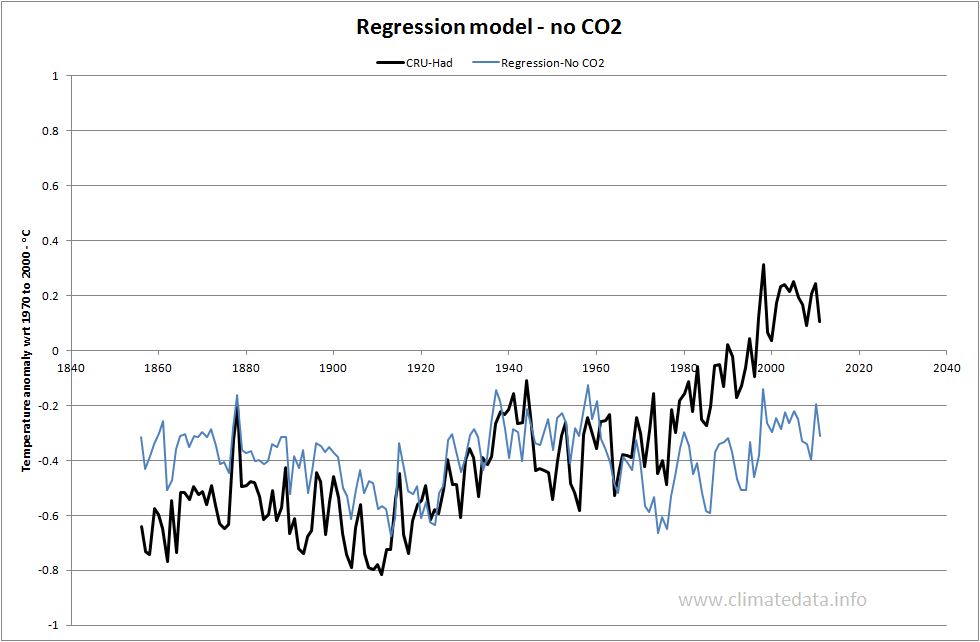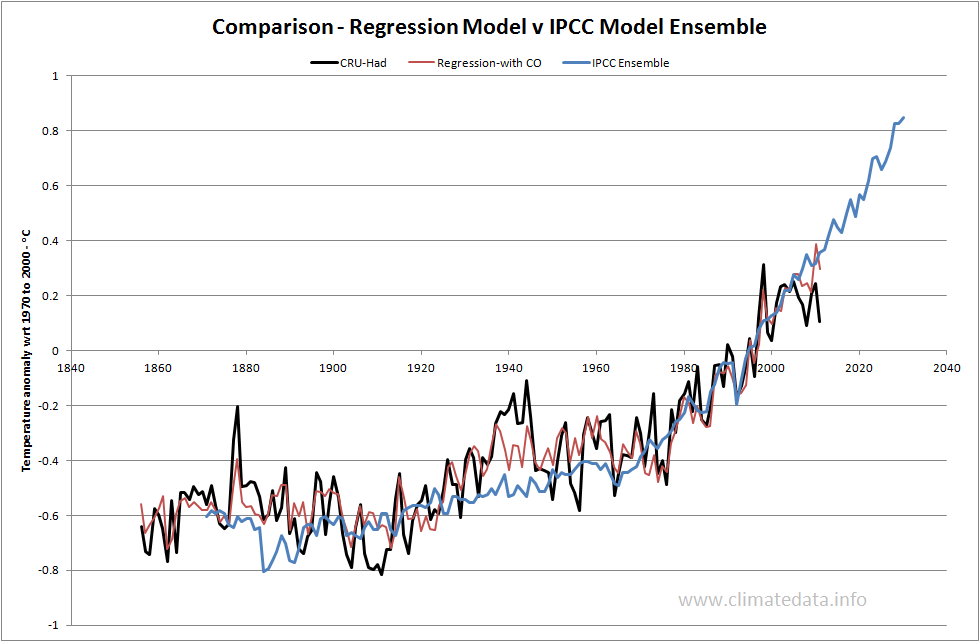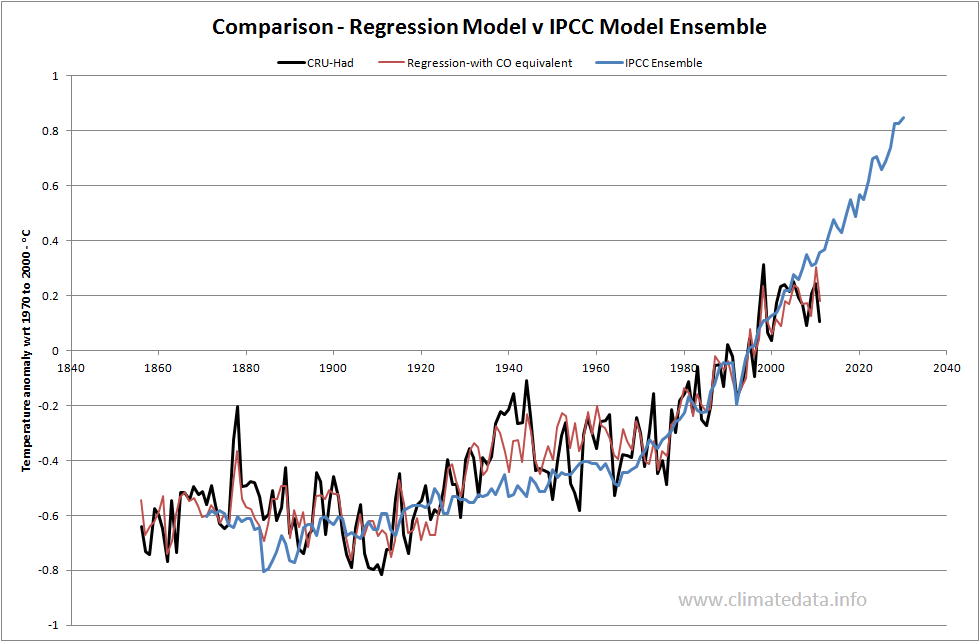# A SIMPLE REGRESSION MODEL - WITH AND WITHOUT CO2 EQUIVALENT

All scientists are well aware of the mantra “Correlation does not imply causation” and people have identified examples to illustrate this. Two spurious correlations I remember were one between the number of missionaries sent to Jamaica and the number of illegitimate babies born the following year, and another between the number of nesting storks and the number of babies born in some German towns. That said, correlation can indicate possible links; cancer and smoking being a case in point. When a physical explanation for a phenomenon is known then regression can also indicate the relative importance of different factors.

Scafetta’s models discussed at scepticalscience.com are examples of regression models which assume that global temperature can be explained by astronomical and climatic cycles superimposed on an underlying but unexplained trend. With some justification they have been dubbed ‘climastrology’.

To look at this in a bit more detail I’ve been playing around with two simple regression models. The first used three parameters: sunspots (SS - as a proxy for solar radiation), optical thickness (OT - to represent aerosols from volcanoes and elsewhere) and Atlantic Multidecadal Oscillation (AMO, as a representative climatic cycle). This is similar to the model Foster and Rahmstorf used to determine the underlying temperature trend from 1979 to 2010 with AMO replacing the El Nino index. The most questionable parameter in both cases is the use of natural climatic oscillations as independent variables. The regression parameters were calculated using the HadCRU3 temperature base and the LINEST function in Excel.

The resulting equation was:

Temperature = 0.62 * AMO + 0.00098*SS + 0.092*OT – 0.44

The equation has an r2 value of 0.19 and a standard error estimate of 0.24 °C. One anomaly is that the coefficient for the Optical Thickness is positive – implying that volcanoes would increase temperature! From these statistics you would not expect the agreement to be very good and it is not.I then tried a four parameter regression, adding CO2. This gave the following equation.

Temperature = 0.49 * AMO + 0.00035 * SS -0.15 * OT + 0.0082 * CO2 - 2.98
I
n this case the r2 value is 0.89 and the standard error of estimate is 0.091 °C. The coefficients are now in the right direction. For comparison I have also plotted the ensemble of IPCC climate models in this case the standard error of estimate is 0.14, rather worse than the regression model.The regression model does as well as the IPCC ensemble in places where the IPCC ensemble performs well (the increase from 1970 to 2000)and the regression model does better in places where the IPCC ensemble is known to underperform ( the increase from 1910 to 1945, the slight decline from 1945 to 1970 and the levelling off from 2000 to 2011). It is clear that the IPCC models will improve dramatically when they are able to simulate climate oscillations.

Ater I had developed this model I remembered that CO2 is not the only greenhouse gas so I modified it to use CO2 equivalent (CO2Eqv). The data were taken mainly from the GISS site (http://data.giss.nasa.gov/modelforce/ghgases//GHGs.1850-2000.txt) updated to 2011. The new model gave the following regression:

Temperature = 0.55 * AMO + 0.000049 * SS -0.35 * OT + 0.0177 * CO2Eqv - 2.98

In this case the r2 value is 0.90 and the standard error of estimate is 0.087 °C - a slight improvement. One intersting difference between the models is that in this model the influence of sunspots is considerably reduced and that of optical thickness (aerosols) is increased.A final point – which may or may not be significant but I throw it in for fun. The coefficient for CO2Eqv is 0.0177. During that period CO2Eqv increased from 537.4 to 956.8 ppm and accounted for 0.74 °C of warming. The ratio of the increase of CO2Eqv was 1.78. This implies a CO2Eqv sensitivity of 0.89 °C for a CO2Eqv doubling (0.74 * log2)/log(1.78)), a figure close to most estimates. Given the warning about not reading too much into correlations with which I started this piece it should be treated with caution.

The modelling using CO2 equivalent was added after the initial post. The final figure with corrected labelling was replaced on 4 March.